# Design of Robust Controller for Nonlinear Systems

Design of Robust Controller for Nonlinear Systems
Control and Systems Engineering Department, University of Technology, Baghdad 10001, Iraq

Corresponding Author Email:
Page:
581-589
|
DOI:
https://doi.org/10.18280/mmep.100227
13 November 2022
|
Revised:
1 March 2023
|
Accepted:
12 March 2023
|
Available online:
28 April 2023
| Citation

OPEN ACCESS

Abstract:

In this study, a nonlinear robust state feedback based on H-infinity controller for nonlinear systems is constructed. On the basis of a proposed cost function, the black hole optimization (BHO) method is employed as a successful optimization approach to discover the optimum parameters with relation to the proposed controller. Solving the H-infinity algebraic Riccati equation yields the recommended controller gain matrix. To demonstrate the efficacy of the suggested controller, two categories of nonlinear systems are provided as case studies. Lastly, the simulation findings reveal that the proposed nonlinear controller is capable and enhances the performance and stability of the nonlinear systems.

Keywords:

nonlinear systems, robust control, H-infinity, optimal control

1. Introduction

Nonlinear systems have been recognized as the most important subject in control field for the previous several decades. They are crucial because of their scientific and engineering applications . Nonlinear phenomena are commonly used to describe the real-world system behavior. Maintaining acceptable stability margins and needed performance parameters in relation to closed-loop systems is difficult because of the substantial nonlinearity and uncertainty of these systems. As a result, robust control approaches are necessary to build nonlinear systems controllers that fulfill the requirements for stability and performance under uncertainty and nonlinearity .

A major difficulty in control theory is the construction of strong feedback controllers. These controllers may achieve asymptotically tracking and robustness in the presence of system disturbance and uncertainty . The feedback control theory includes several structure options for feedback as well as various feedback loops. The state feedback control is a sort of feedback control wherein the feedback is accessible for all system states . The H-infinity control, which is considered one of the state feedback control, is the most prevalent and successful method in robust control theory in order to reject a disturbance and compensating uncertainties and nonlinearities in the system. It gives excellent performance and stability [5-8].

Previous studies have concentrated on a range of robust feedback control design methodologies, for example, the development of H-infinity state feedback control depending on a nearly linearized model [9, 10], based on lyapunove theory, the robust feedback linearization for nonlinear process control is built , the H-infinity loop - shaping strategy [12, 13], and a robust method of control based on linear & bilinear matrix inequalities, (LMIs) and (BMIs) [14, 15].

Each of the previous research offered somewhat difficult procedure for creating a nonlinear system controller that are particular with relation to the kind of system, which encouraged us to try to use another procedure design method which recommends a simpler and more universal approach.

A proposed full state feedback controller is built in this research to realize the stability for nonlinear systems as well as achieve a desired performance. To determine the best settings for the proposed nonlinear controller, the Black Hole optimization (BHO) approach is utilized. The suggested controller in this study may successfully improve the nonlinear systems behaviour of fixed coefficients.

The rest of this article is organized as following: The black hole optimization (BHO) is detailed in the part "Black Hole Optimization (BHO) method". The concept of the control design based on state feedback control and H-infinity control theory for a nonlinear system is described in the "Controller Design" part. The suggested controller’s parameters that will be adjusted by the (BHO) to get the best values for them were presented in the "Controller Parameters Tuning" part. Two categories of nonlinear systems are offered as case studies in the "Illustrative Examples" part to show the efficacy of the suggested controller. Conclusions are provided in the "Conclusion" section.

2. Black Hole Optimization Method

The BHO is a relatively new optimization approach that is widely utilized to discover the most suitable solution to a combinatorial optimization problem . It is classified as a metaheuristic (population-based) optimization method since it employs a particular trade-off for randomization versus local search to be able to get a solution that is optimal or almost perfect . Local search is a popular approach for locating superior solutions to complex or challenging combinatorial optimization issues in a reasonable period of time. In addition, it is a method of iterative search for varying neighboring solutions in order to improve on current ones [16-18].

This approach will be used in this study to make adjustments to a number of significant parameters through the course of building the controller of a nonlinear system, resulting in the optimal values for these parameters which yield the best stability and performances.

For this method, A population of possible solutions (stars) is generated randomly from of the points situated inside the search space. Following startup, the fitness values of the population are assessed, and the most qualified candidate (having the greatest fitness value) is chosen to represent the black hole, however the remaining stars become the regular stars. As a result, the black hole begins to attract stars from all directions around it, which move toward the black hole [16-19]. The formal migration of stars toward a black hole is expressed as in researches [17-19]:

\begin{aligned} x_i(t+1)= & x_i(t)+\operatorname{rand}\left(x_{B H}-x_i(t)\right) \\ & i=1,2,3, \ldots, N\end{aligned}                          (1)

where, xi(t+1) and xi(t) represent the coordinates for the ith star on the iteration (t+1) and (t), respectively. (rand) is a number ranging from 0 to 1 that is created at random. The location of the black hole inside the search space is denoted by (xBH). (N) represents the number of potential solutions (stars).

A star shifts its position as it approaches the black hole, if the star’s fitness value surpasses the black hole value, it is selected as the black hole. After then, the procedure is repeated also with the black hole within the new location, and stars start to gravitate toward that new black hole. consequently, traveling stars approaching to black hole have a probability of passing through the event horizon. every candidate solution (star) that exceeds the event horizon of a black hole will be devoured by the black hole. Then, after the swallowed star, a new star is formed and spread throughout in the search space at random. The goal of that construct is to maintain a constant number for candidate solutions. The following iteration begins when the stars have all shifted . The following formula is used to calculate the event horizon radius (R) [17-19]:

$R=\frac{f_{B H}}{\sum_{i=1}^N f_i}$                                   (2)

where, fBH is the fitness value of the black hole, N denotes the number of solutions that are possible (stars) and fi represents the fitness value of the ith star. Whenever the separation of a star and a black hole is less than a particular radius (R), this star has been swallowed via the black hole.

The BH algorithm's feasibility is determined by determining the best parameters for the proposed controllers. At first, specify the populations size and the dimensions of the problem, as well as the controller parameters to be optimized. In this method, this parameter will be represented by the stars, and the most effective solutions being represented by the black holes. The black holes then begin to engulf neighboring stars during the previously indicated stages. The previously optimized parameters (best solutions or the black holes) are then determined and concurrently introduced to the controlled system to compute the detected error and control action. As a result, the measured error and control action are utilized to determine the cost function, which is then compared to the prior cost during every iteration to acquire the better cost and afterward the ideal parameters. Lastly, after a specific number of iterations, this procedure is repeated until the ideal parameters are determined .

The use of the black hole optimization approach has two advantages. First, it has a basic framework that is easy to implement. Second, no issues there are with regard to parameter adjustments .

3. Controller Design

This part describes the steps design of suggested controller for systems of the model [20, 21]:

$\begin{gathered}\dot{x}(t)=A x(t)+B_1 d(t)+B_2 u(t) \\ e(t)=C_1 x(t)+D_{12} u(t) \\ y(t)=C_2 x(t)\end{gathered}$                              （3）

where, $x(t) \in \mathcal{R}^n$ represents state vector of the system, $d(t) \in \mathcal{R}^m$ denotes the exogenous disturbance, $u(t) \in \mathcal{R}^l$ depicts the control input, $e(t) \in \mathcal{R}^q$  denotes the controlled output, $y \in \mathcal{R}^p$ represents the output as measured, which is supposed to represent the state vector accessible for feedback, $A \in \mathcal{R}^{n \times n}, B_1 \in \mathcal{R}^{n \times m}$ and $B_2 \in \mathcal{R}^{n \times l}, C_1 \in \mathcal{R}^{q \times n}$ denotes the system state control weight matrix, $D_{12} \in \mathcal{R}^{q \times l}$ represent the control input regulation weight matrix, $C_2 \in \mathcal{R}^{p \times n}$  is aweight matrix of the output.

Figure 1 depicts the conventional setup of the complete state feedback H-infinity control, while Kc is the controller gain matrix and M stands for the augmented system matrix .

$M=\left[\begin{array}{ccc}A & B_1 & B_2 \\ C_1 & D_{11} & D_{12} \\ C_2 & D_{21} & D_{22}\end{array}\right]$                                  (4)

## 1.pngFigure 1. The structure of H-infinity full state feedback control

Feedback should be possible for all states of system in order to develop a complete state feedback H-infinity control. This means that C2=I.D11, D21 and D22 are all equal to zero. As a result, the augmented system matrix M is:

$M=\left[\begin{array}{ccc}A & B_1 & B_2 \\ C_1 & 0 & D_{12} \\ I & 0 & 0\end{array}\right]$                   (5)

The suggested controller design must make the following assumptions:

1. The pairings (A, B1) & (A, B2) are supposed to be controllable or, at the very least, stabilizable.

2. The pairing (C1, A) is supposed to be observable or, at the very least, detectable.

3. $C_1^T D_{12}=0 \& D_{12}^T D_{12}=I$.

It is important to remember that the nonlinear system must be changed to the form described in Eq. (3) utilizing a state variable transformation technique before being controlled in this approach. This transformation arranges nonlinear terms as well uncertainty (bad terms) at the identical channel as the control law u (achieving the matching requirement). In this circumstance, the controller may compensate for the unsatisfactory terms. The diffeomorphism mapping is the suitable transformation for this object:

$\phi: \mathrm{D} \rightarrow \mathcal{R}^n$ this converts the system from x to z space .

$z=\phi(x)$                         (6)

The map $\phi$ must be invertable in order to:

$x=\phi^{-1}(z)$                        (7)

The z-space origin point of the modified system equals the point of the initial system .

$\phi(0)=0$                             (8)

The above mapping transforms our nonlinear system into the form shown below :

$\dot{z}(t)=A z(t)+B_1 d(t)+B_2 u(t)$                            (9)

The goal of the control challenge is to discover the optimum control law u*, which is state dependent, that ensures that the closed-loop transfer function’s (Ted) infinite norm is smaller than a certain value of γ as :

$\left\|T_{e d}(s)\right\|_{\infty}<\gamma$                         (10)

where, γ denotes the upper constraint on the amplitude of the disturbance and perturbation which could be eliminated through the control action. The requirement in Eq. (10) denotes that :

$\underset{u}{\inf} \underset{d}{\sup} J(u, d)<\infty$                         (11)

where,

$J(u, d)=\int_0^{\infty}\left(e^T e-\gamma^2 d^T d\right) d t$                                 (12)

The disturbance d(t) attempts to maximize the cost function J(t), whereas the control signal u(t) intends to minimize it. As a result, the physical connotation of this relationship is that the control action and also the disturbance are in competition with one other within infinmum (inf) and supremum (sup).

Let the structure of the suggested optimal controller and worst-case disturbance be as follows :

$d(t)=K_d x(t)$                            (13)

And

$u(t)=K_c x(t)$                                  (14)

By substituting Eq. (14) into Eq. (3) results in:

$e(t)=\left(C_1+D_{12} k_c\right) x(t)$                                  (15)

Utilising assumption 3, we get:

$e^T e=x^T\left(C_1^T C_1+K_C^T K_c\right) x$                          (16)

Therefore,

$J=\int_0^{\infty} x^T\left(C_1^T C_1+K_c^T K_c-\gamma^2 K_d^T K_d\right) x d t$                                 （17）

Substituting Eqns. (13) and (14) into Eq. (3) results in:

$\dot{x}=\left(A+B_1 K_d+B_2 K_c\right) x$                                    （18）

from the cost function in Eq. (17), can let:

$Q=\left(C_1^T C_1+K_c^T K_c-\gamma^2 K_d^T K_d\right)$                             （19）

where, should be positive definite matrix.

By the optimal control law, the system acquired by Eq. (18) is considered to be stable. Based on this assumption, let us develop:

$V(x)=x^T P x$                                                 (20)

$\dot{V}(x)=-x^T Q x$                                  (21)

V(x) represents a Lyapunov function with a positive definite. Eq. (19) would then substituted in Eq. (21) to obtain the best cost function:

$\dot{V}(x)=-x^T\left(C_1^T C_1+K_c^T K_c-\gamma^2 K_d^T K_d\right) x$                                       (22)

$x^T\left(C_1^T C_1+K_c^T K_c-\gamma^2 K_d^T K_d\right) x=-\frac{d}{d t} x^T P x$                                    (23)

By integrating on each side of Eq. (23) from 0 to $\infty$ gives:

$\begin{gathered}\int_0^{\infty} x^T\left(C_1^T C_1+K_c^T K_c-\gamma^2 K_d^T K_d\right) x d t= \\ \int_0^{\infty}-\frac{d}{d t} x^T P x d t\end{gathered}$                    (24)

$J=-x(\infty)^T P x(\infty)-\left(-x(0)^T P x(0)\right)$                                  (25)

Because the system in Eq. (18) should be stable according to the control law, x(∞)=0. As a result, the optimal cost function is:

$J^*=-x(0)^T P x(0)$                                  (26)

The solution to the following Lyapunov equation is represented by the positive definite matrix P:

$\begin{gathered}\left(A+B_1 K_d+B_2 K_c\right)^T P+P\left(A+B_1 K_d+B_2 K_c\right)=-Q\end{gathered}$                                   （27）

$\begin{array}{r}\left(A+B_1 K_d+B_2 K_c\right)^T P+P\left(A+B_1 K_d+B_2 K_c\right) =-\left(C_1^T C_1+K_c^T K_c-\gamma^2 K_d^T K_d\right)\end{array}$                                       （28）

\begin{aligned} & \left(A+B_1 K_d+B_2 K_c\right)^T P+P\left(A+B_1 K_d+B_2 K_c\right) +\left(C_1^T C_1+K_c^T K_c-\gamma^2 K_d^T K_d\right)=0\end{aligned}                      (29)

To obtain the optimum control law, Eq. (29) must be derived with respect to Kc and set $\partial P / \partial K_{c_{i j}}=0$, we get:

$K_C=-B_2^T P$                                      (30)

As a result,

$u^*=K_c x=-B_2^T P x$                                     (31)

Similarly, we may get the applied worst-case disturbance via deriving the Lyapunov equation with respect to Kd and setting $\partial P / \partial K_{d_{i j}}=0$, we get:

$K_d=\frac{1}{\gamma^2} B_1^T P$                                    (32)

And

$d^*=K_d x=\frac{1}{\gamma^2} B_1^T P x$                                            (33)

And at optimum control condition and worst-case disturbance, we have the Lyapunov equation:

$P A+A^T P+C_1^T C_1-P\left(B_2 B_2^T-\frac{1}{\gamma^2} B_1 B_1^T\right) P=0$                                       (34)

This formula is called H-infinity algebraic riccati equation (HIARE).

The provision $\left\|T_{e d}(s)\right\|_{\infty}<\gamma$ is satisfied and provided:

1. $u^*=K_c x=-B_2^T P x$
2. P>0
3. The matrix A+B1Kd+B2Kc is stable, which means that the matrix A+B2Kc is asymptotically stable as well.
4. Controller Parameters Tuning

The black hole optimization technique is used offline, which means that an algorithm that works offline is given the entirety of issue data from the beginning and is necessary to develop a solution to a current problem, as well as to collect the proposed controller needed parameters. The black hole optimization method is straightforward and it was conducted to discover the optimal values for the components of matrix C1 (Eq. (3)) as well as the optimal value of γ (Eq. (34)) that fulfill the necessary robustness in stability and performance. Figure 2 depicts the proposed controller block diagram with the BHO algorithm. The goal of the BHO issue is to determine the best controller from the search space which minimizes the proposed cost function and meets Eq. (34). The following parameters were utilized to implement the robust controller design utilizing the black hole optimization (BHO):

1. The members to be gained are: c11, c12, …, cnn and γ.

2. the population size is fixed at 50.

3. The maximum number of iterations is set to 100.

The controller parameters are tuned subject to the following cost function:

$J=\int_0^{t_f} e^2(t) d t+\int_0^{t_f} u^2(t) d t$                                       (35)

where, e represents the error, tf represents the final time and u represents the control signal. The cost function in Eq. (35) is used to guarantee a desirable time response specification in addition to the robustness which was guarantee by applying the H-infinity control.

## 2.png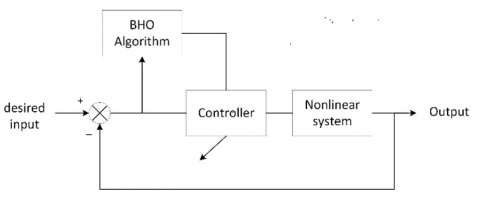Figure 2. The proposed controller’s block diagram using BHO

5. Illustrative Examples

The nonlinear system that must be controlled is:

$\begin{gathered}\dot{x}_1=x_2 \\ \dot{x}_2=\sin x_1-u \times \cos x_1 \\ y=x_1\end{gathered}$                                     (36)

Figure 3 shows the stages of designing the proposed controller for the system described in Eq. (36).

## 3.png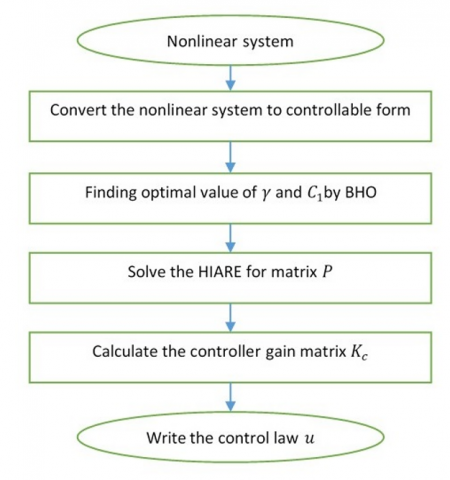Figure 3. The controller designing stages for example 1

## 4.png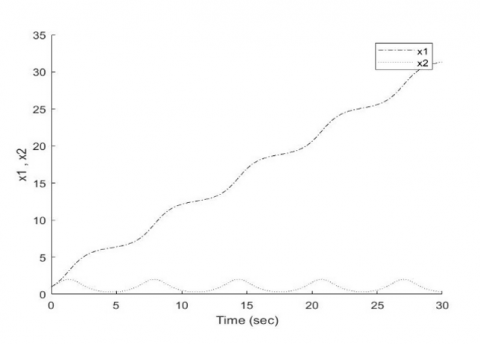Figure 4. Open-loop system time response prior using the proposed controller

## 5.png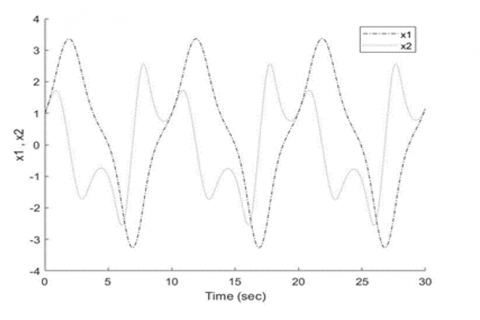Figure 5. Closed-loop system time response prior using the proposed controller

Figures 4 and 5 depict the open and closed loops nonlinear system time responses features respectively prior to applying the suggested controller. In open-loop, our system is clearly unstable, but in closed-loop, it is critical stable, thus to stabilize the system as well as obtain the desired performance, a controller must be built.

There is no requirement for employ the diffeomophism mapping for this system since all of the nonlinear parts are situated within the same control channel u (the matching condition is met). According to the system equation (Eq. (35)), the factor of u is a nonlinear variable component of the state x1. As a result, the following procedure is necessary to convert the system equation (Eq. (35)) to the conventional controllable structure as Eq. (3):

$v=-u \times \cos x_1$                            (37)

where, v denotes the virtualized linear state feedback controller, that is:

$v=K_C x=K_1 x_1+K_2 x_2$                                   (38)

Then the actual controller u is:

$u=-\frac{v}{\cos x_1}=-\frac{K_c x}{\cos x_1}=\frac{-K_1 x_1-K_2 x_2}{\cos x_1}$                                         (39)

The state equation for the system that results is:

$\dot{x}(t)=\left[\begin{array}{ll}0 & 1 \\ 0 & 0\end{array}\right] x(t)+\left[\begin{array}{l}0 \\ 1\end{array}\right] d(t)+\left[\begin{array}{l}0 \\ 1\end{array}\right] v(t)$                                 (40)

where,

$d(t)=\sin x_1$                                        (41)

The optimal control law then was determined by using the (BHO) method. Table 1 shows the BHO algorithm optimization setting. Table 2 includes the optimized parameter’s ideal values as well as their limits.

Table 1. The BHO algorithm setting (1st Example)

 Optimisation settings Value Problem dimensions (number of parameters) 5 Population size 50 Iterations count 100 Number of rounds 1

Table 2. The optimized parameters’ optimal values and bounds (1st Example)

 Optimized parameter Least bound Upper bound Optimum value γ 1 10 7.1288 c11 0 1000 24.7304 c12 0 1000 44.8355 c13 0 1000 83.699 c14 0 1000 93.5115

## 6.png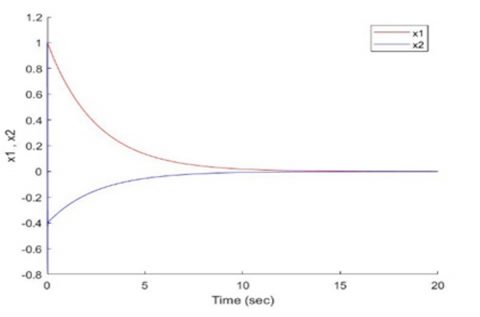Figure 6. Stabilization features for the states of the nonlinear system

## 7.png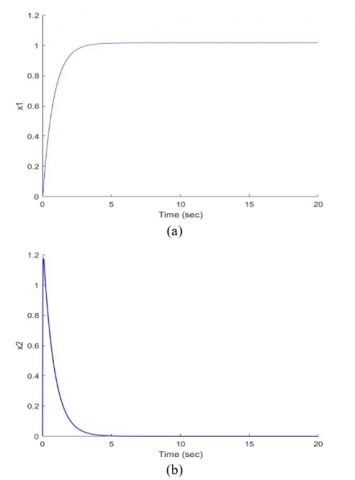Figure 7. (a) Tracking properties of the controlled nonlinear system; (b) x2 behaviour

Following that, the optimal values will be used to solve the H-infinity algebraic riccati problem (Eq. (34)) to get the matrix P of stabilizing positive definiteness as follows:

$P=10^3\left[\begin{array}{ll}0.1843 & 0.0517 \\ 0.0517 & 0.1272\end{array}\right]$                                                     (42)

The state feedback controller’s gain matrix was then calculated using Eq. (30), as shown below:

$K_c=\left[\begin{array}{ll}-51.7 & -127.2\end{array}\right]$                                      (43)

Thus, the control law becomes:

$u=\frac{51.7 x_1+127.2 x_2}{\cos x_1}$                                                     (44)

Figure 6 demonstrates the nonlinear system time response behaviour after applying the suggested controller and achieving the stabilization. Figure 7 depicts the output tracking for the entry of a unit step reference and the behaviour of state x2 which is stable. Figure 8 shows the magnitude of error between input and output signals which is acceptable. Figure 9 depicts the control action behavior. It appears that the suggested controller can successfully stabilizes the nonlinear system while maintaining acceptable performance.

## 8.png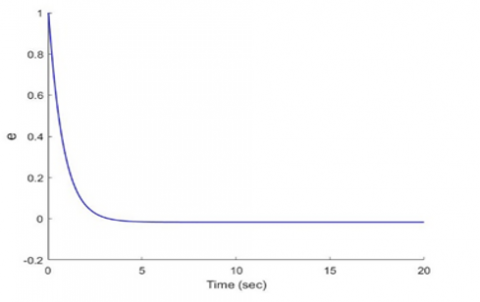Figure 8. Error signal properties

## 9.png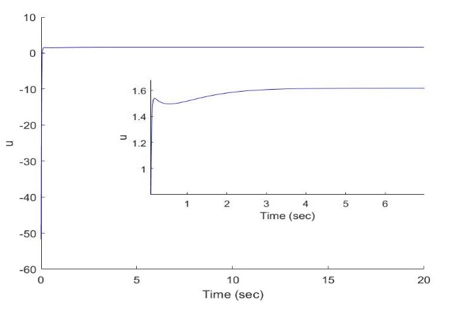Figure 9. The resultant control action

5.2 Example (2)

Considering the nonlinear system that must be controlled:

$\begin{gathered}\dot{x}_1=\tan x_1+x_2 \\ \dot{x}_2=x_1+u \\ y=x_1\end{gathered}$                                    (45)

Figure 10 shows the stages of designing the proposed controller for the system described in Eq. (45).

## 10.pngFigure 10. The controller designing stages for example 2

## 11.pngFigure 11. Open-loop system time response prior using the proposed controller

## 12.pngFigure 12. Closed-loop system time response prior using the proposed controller

Figures 11 and 12 depict the open and closed loops nonlinear system time responses features respectively prior to applying the suggested controller.

The system is clearly unstable in both open and closed loops, thus to stabilize the system as well as obtain the desired performance, a controller must be built. Since the nonlinear term (tanx1) is not placed on the identical channel with the control action u (matching requirement not met), the diffeomorphism mapping for Eq. (6) is required to turn the nonlinear system to its controllable standard structure such as Eq. (9). The state variable transformation shown below is used to carry out the mapping:

$z_1(t)=x_1(t), z_2(t)=\dot{z}_1(t)=\dot{x}_1(t)=\tan x_1+x_2$                                   (46)

Thus, the transformed state equation becomes:

$\begin{gathered}\dot{z}_1(t)=z_2(t) \\ \dot{z}_2(t)=z_1+d(t)+u(t)\end{gathered}$                                               (47)

where,

$d(t)=z_2 \sec ^2 z_1$                                             (48)

The system in z-space then becomes:

$\left[\begin{array}{l}\dot{z}_1(t) \\ \dot{z}_2(t)\end{array}\right]=\left[\begin{array}{ll}0 & 1 \\ 1 & 0\end{array}\right]\left[\begin{array}{l}z_1(t) \\ z_2(t)\end{array}\right]+\left[\begin{array}{l}0 \\ 1\end{array}\right] d(t)+\left[\begin{array}{l}0 \\ 1\end{array}\right] u(t)$                                            (49)

The optimal control law then was determined by using the (BHO) method. Table 3 shows the BHO algorithm optimization setting. Table 4 includes the optimized parameter’s ideal values as well as their bounds.

Table 3. The BHO algorithm settings (2nd Example)

 Optimisation settings Value Problem dimensions (a number of parameters) 5 Population size 50 Iterations count 100 Number of rounds 1

Table 4. The optimized parameters’ optimal values and bounds (2nd Example)

 Optimized parameter Least bound Upper bound Optimum value γ 1 10 6.7995 c11 0 1000 69.0007 c12 0 1000 41.3458 c21 0 1000 81.6022 c22 0 1000 95.2433

Following that, the optimal values will be used to solve the H-infinity algebraic riccati problem (Eq. (34)) to get the matrix P of stabilizing positive definiteness as follows:

$P=10^3\left[\begin{array}{ll}0.5735 & 0.0823 \\ 0.0823 & 0.1274\end{array}\right]$                                (50)

The state feedback controller’s gain matrix was then calculated using Eq. (30), as shown below:

$K_c=\left[\begin{array}{ll}-82.3554 & -127.4602\end{array}\right]$                                            (51)

As a result, the optimum control law in z-space becomes:

$u=-82.3554 z_1-127.4602 z_2$                                       (52)

The control law is now being transformed from z-space to x-space:

$u=-82.3554 x_1-127.4602 \tan x_1-127.4602 x_2$                                           (53)

## 13.png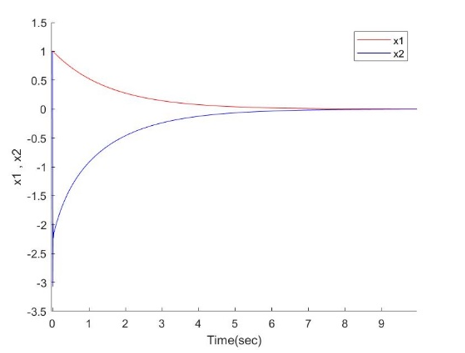Figure 13. Nonlinear system states’ stabilization qualities

## 14.pngFigure 14. (a) Tracking properties of the controlled nonlinear system; (b) x2 behaviour

Figures 13 and 14(a) illustrate that, despite the existence of disturbance, the suggested controller succeeded in providing nonlinear system stability and tracking performance efficiency with little tracking error as well as quick converging to the reference input. Figure 14(b) shows the behaviour of state x2 which is stable. Figure 15 shows the error magnitude between input and output signals which is acceptable. Figure 16 depicts the permissible behavior of the control action that has been executed.

## 15.png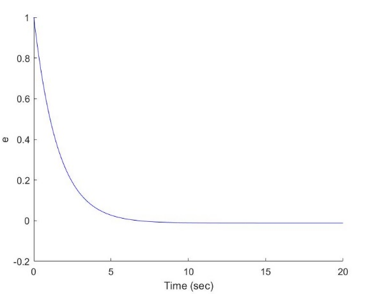Figure 15. Error signal properties

## 16.pngFigure 16. The resultant control action

6. Conclusion

A new robust optimal controller premised on the H-infinity approach has been developed in this paper for two different kinds of nonlinear systems: the first one meets the condition for matching the nonlinear terms (disturbances) and control law, while the latter does not. The suggested controller architecture achieved excellent tracking performance and stability in both scenarios. The systems were asymptotically stable after applying the suggested controller. To find the optimal values for the controller parameters, the black hole optimization approach was utilized. This work has contributed by offering a simple design technique algorithm of the suggested controller for a broad variety of nonlinear systems, implying that it is a generic algorithm, whereas earlier studies exhibited a difficult design algorithm that was specific to the sort of nonlinear system. Finally, whenever the coefficients of the system are uncertain, another controller design is required.

References

 Christos, K.V. (2017). Nonlinear Systems: Design, Applications and Analysis. SIAM.

 Ali, H.I. (2018). Swarm Intelligence to Robust Control Design. United Scholars Publications, USA.

 Thabet, A., Frej, G.B.H., Gasmi, N., Metoui, B. (2020). Real time stabilization of Lipschitz nonlinear systems with nonlinear output. Journal Européen des Systèmes Automatisés, 53(4): 493-498. https://doi.org/10.18280/jesa.530407

 Mhmood, A.H., Ali, H.I. (2021). Optimal H-infinity integral dynamic state feedback model reference controller design for nonlinear systems. Arabian Journal for Science and Engineering, 46(10): 10171-10184. https://doi.org/10.1007/s13369-021-05447-4

 Ali, H.I., Hadi, M.A. (2020). Optimal nonlinear controller design for different classes of nonlinear systems using black hole optimization method. Arabian Journal for Science and Engineering, 45(8): 7033-7053. https://doi.org/10.1007/s13369-020-04650-z

 Yadav, A.K., Pathak, P.K., Gaur, P. (2020). Robust control and stability analysis of computerized numeric controlled machine tool under parametric uncertainty. Journal Européen des Systèmes Automatisés, 53(5): 661-670. https://doi.org/10.18280/jesa.530509

 Ali, H.I., Abdulridha, A.J. (2018). H-infinity based full state feedback controller design for human swing leg. Engineering and Technology Journal, 36(3A): 350-357. https://doi.org/10.30684/etj.36.3A.15

 Ali, H.I., Mhmood, A.H. (2021). Nonlinear H-infinity model reference controller design. International Review of Automatic Control (IREACO), 14(1): 39-50.

 Rigatos, G., Siano, P., Abbaszadeh, M., Ademi, S. (2017). Nonlinear H-infinity control for the rotary pendulum. 2017 11th International Workshop on Robot Motion and Control (RoMoCo), Wasowo Palace, Poland, pp. 217-222. https://doi.org/10.1109/RoMoCo.2017.8003916

 Rigatos, G., Siano, P. (2015). A new nonlinear H-infinity feedback control approach to the problem of autonomous robot navigation. Intelligent Industrial Systems, 1(3): 179-186. https://doi.org/10.1007/s40903-015-0021-x

 de Jesús Rubio, J. (2018). Robust feedback linearization for nonlinear processes control. ISA Transactions, 74: 155-164. https://doi.org/10.1016/j.isatra.2018.01.017

 Jones, B.L., Heins, P.H., Kerrigan, E.C., Morrison, J.F., Sharma, A.S. (2015). Modelling for robust feedback control of fluid flows. Journal of Fluid Mechanics, 769: 687-722. https://doi.org/10.1017/jfm.2015.84

 Flinois, T.L.B., Morgans, A.S. (2016). Feedback control of unstable flows: A direct modelling approach using the Eigensystem Realisation Algorithm. Journal of Fluid Mechanics, 793: 41-78. https://doi.org/10.1017/jfm.2016.111

 Gritli, H., Belghith, S. (2018). Robust feedback control of the underactuated Inertia Wheel Inverted Pendulum under parametric uncertainties and subject to external disturbances: LMI formulation. Journal of the Franklin Institute, 355(18): 9150-9191. https://doi.org/10.1016/j.jfranklin.2017.01.035

 Gritli, H. (2019). Robust master-slave synchronization of chaos in a one-sided 1-DoF impact mechanical oscillator subject to parametric uncertainties and disturbances. Mechanism and Machine Theory, 142: 103610. https://doi.org/10.1016/j.mechmachtheory.2019.103610

 Kumar, S., Datta, D., Singh, S.K. (2015). Black hole algorithm and its applications. In: Azar, A., Vaidyanathan, S. (eds) Computational Intelligence Applications in Modeling and Control. Studies in Computational Intelligence, vol 575. Springer, Cham. https://doi.org/10.1007/978-3-319-11017-2_7

 Farahmandian, M., Hatamlou, A. (2015). Solving optimization problems using black hole algorithm. Journal of Advanced Computer Science & Technology, 4(1): 68-74. https://doi.org/10.14419/jacst.v4i1.4094

 Hatamlou, A. (2013). Black hole: A new heuristic optimization approach for data clustering. Information Sciences, 222: 175-184. https://doi.org/10.1016/j.ins.2012.08.023

 Ibrahim, I.H., Ali, H.I. (2021). Quantitative PID controller design using Black Hole optimization for ball and beam system. Iraqi Journal of Computers, Communications, Control and Systems Engineering, 21(3): 65-75. http://dx.doi.org/https://doi.org/10.33103/uot.ijccce.21.3.6

 Shareef, Z.M., Ali, H.I. (2018). Full state feedback H2 and H-infinity controllers design for a two wheeled inverted pendulum system. Engineering and Technology Journal, 36(10): 1110-1121. https://doi.org/10.30684/etj.36.10A.12

 Sinha, A. (2007). Linear Systems: Optimal and Robust Control. CRC Press of Taylor & Francis Group, New York.

 Khalil, H.K. (2002). Nonlinear Systems. 3rd edition. Prentice Hall, Upper Saddle River, New Jersey.